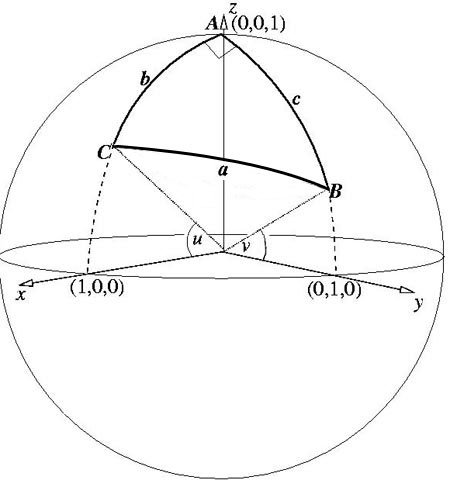#### You may also likeA quadrilateral changes shape with the edge lengths constant. Show the scalar product of the diagonals is constant. If the diagonals are perpendicular in one position are they always perpendicular?As a quadrilateral Q is deformed (keeping the edge lengths constnt) the diagonals and the angle X between them change. Prove that the area of Q is proportional to tanX.### Air Routes

Find the distance of the shortest air route at an altitude of 6000 metres between London and Cape Town given the latitudes and longitudes. A simple application of scalar products of vectors.

# Pythagoras on a Sphere

##### Age 16 to 18 Challenge Level:

 All angles are in radians. (1) Without loss of generality take coordinate axes so that $A$ is the point$(0,0,1)$, the xz-plane contains the point $C$ and the yz-plane contains the point $B$. (2) Thinking of $A$ as the North Pole then $C$ has latitude $u$ and longitude 0 and $B$ has latitude $v$ and longitude $\pi/2$. (3) Find the 3D coordinates of $B$ and $C$. Where the origin O is the centre of the sphere ${\bf OA, OB}$ and ${\bf OC}$ are vectors of unit length. (4) Use scalar products and vectors ${\bf OA, OB}$ and ${\bf OC}$ to find the lengths of the arcs $AB, BC$ and $CA$ in terms of $u$ and $v$. The required result follows.